## Example Questions

### Example Question #1 : Solving Systems Of Equations In Word Problems

Blin and Alex raised a total of $240,000 for a charity. If Blin raised$60,000 more than Alex did, how much money did Blin raise?

$180,000$120,000

$150,000$100,000

$150,000 Explanation: This problem provides you with a classic SAT Systems of Equations setup. You're given the sum of two entities - here that's Blin's Total + Alex's Total =$240,000 - and a relationship between those two entities. Here that's Blin raised $60,000 more than Alex did. The sum equation is generally straightforward; you can express that as B + A = 240,000. For the relationship equation, you can say that Blin's total is$60,000 more than Alex's, which translates to B = 60,000 + A.

Now you have two equations:

B + A = 240,000

B = 60,000 + A

This structure sets up well for Substitution; you already have B in terms of A, so you can plug in the second equation for the first:

(60,000 + A) + A = 240,000

60,000 + 2A = 240,000

2A = 180,000

A = 90,000

Of course, at this point you need to check whether you solved for the proper variable. The question asks you about Blin's total, not Alex's, so you need to add $60,000 to get to Blin's total of$150,000.  Then check your work: does Blin's total of $150,000 plus Alex's total of$90,000 arrive at the sum we know to be $240,000? It does, so you can confidently choose$150,000.

### Example Question #2 : Solving Systems Of Equations In Word Problems

Judy and Nancy participated in a walk-a-thon to raise money for charity. They combined for 42 total miles between them, with Judy walking twice as many miles as Nancy. How many miles did Nancy walk?

18

28

14

12

14

Explanation:

This problem provides you with a classic SAT Systems of Equations setup: you get a sum equation about a combined total of two entities, and then an equation that gives a relationship between the two. Here you know that Judy and Nancy combined for 42 miles, which you can turn into an equation as:

J + N = 42

Then you're told that Judy walked twice as many miles as Nancy did. That equation is J = 2N (be careful about putting the two in the wrong spot - it's best to think about "what do I have to do to Nancy's total to make it equal Judy's?? You'd have to double it to catch up, so twice Nancy's total is what it takes to equal Judy's --> J = 2N).

Now you can substitute the second equation for the first. If you plug in 2N where the J appears in the first, you have:

2N + N = 42

3N = 42

N = 14

And since this problem asks you for Nancy's total, you have it: Nancy walked 14 miles.

### Example Question #3 : Solving Systems Of Equations In Word Problems

Siblings Tamika and David tracked every hour they spent studying during a particular school year. Combined, they studied for a total of 932 hours, with Tamika studying for 28 more hours than her brother David did. How many hours did Tamika study?

450

480

390

510

480

Explanation:

This problem provides you with two equations, setting up nicely for a Systems of Equations approach. The first equation is about their combined total, and you can set it up as:

T + D = 932

Then the second equation is:

T = D + 28. (you can think of this as "to equal Tamika's total, David would have had to add 28 hours")

One helpful tip on this problem is that while it sets up nicely for a Substitution Method approach (substitute D + 28 for T in the first equation), you want to solve for T, not for D. So you can with one quick algebra step set it up to use the Elimination Method. Just subtract D from both sides in the second equation, and it becomes T - D = 28

Now you can stack your equations and sum them:

T + D = 932

T - D = 28

These sum to:

2T = 960

Divide both sides by 2 and you get T = 480.

### Example Question #21 : Solving Word Problems

Joanna has two dogs, Rover and Spot, who weigh a combined total of 87 pounds. If Rover weighs 9 pounds more than Spot, how many pounds does Spot weigh?

39

48

54

43

39

Explanation:

This problem provides you with two equations, allowing you to use Systems of Equations logic to solve once you've constructed the equations. Often on the SAT, these Systems problems will start by giving you a combined total as this one does. If the two dogs weigh a total of 87 pounds, you can express that as:

R + S = 87 (in which R = Rover's weight and S = Spot's weight)

Then if Rover weighs 9 pounds more than Spot, you have a few choices for how to express that. One is to say that "Rover's weight is 9 pounds more than Spot's weight" and then use the fact that "is" means "equals" so that the words translate directly to the equation: R = 9 + S.  Another way is to see that Rover weighs more, and the difference is 9, meaning that R - S = 9.  The equation with subtraction allows you to go straight into the Elimination Method as you have a + S in the first equation and a -S in the second, so that may be your preferred choice. If you structure it that way, you can stack the equations and sum them:

R + S = 87

R - 9 = 9

Which sums to:

2R = 96

That means that R = 48, but remember in Systems of Equations problems to always check that your algebra has answered the specific question, as a problem with two variables allows you to mistakenly solve for the wrong one! The question asks you about Spot's weight, so you can subtract 9 from 48 to see that Spot weighs 39 pounds.

The snack bar at a certain lake sells slices of pizza in two varieties: vegetarian pizza costs $1.25 per slice, and pepperoni pizza costs$1.50 per slice. If the snack bar sold a total of 20 slices of pizza for a total of $28.25 on a particular day, how many more slices of pepperoni were sold than slices of vegetarian? Possible Answers: 13 10 6 7 Correct answer: 6 Explanation: This problem provides you with the opportunity to set up two equations: the total number of slices of pizza, and the total amount of money. If you use V to represent the number of vegetarian slices and P to represent the number of pepperoni slices, you can express the total number of slices as: V + P = 20 Then for the money, the total revenue will be a combination of how much was made from selling vegetarian slices ($1.25 times the number of vegetarian) and how much was made from selling pepperoni ($1.50 times the number of pepperoni). This equation is then: 1.25V + 1.5P = 28.25 This problem sets up nicely for using the Substitution Method for systems of equations. Since V is likely easier to work with in the second equation (the substituted term is likely easier to multiply by 1.5 than by 1.25), you can leverage the first equation to get P in terms of V: P = 20 - V When you plug this in to the second equation, you get: 1.25V + 1.5(20 - V) = 28.25 This expands to: 1.25V + 30 - 1.5V = 28.25 Combine like terms to get: -0.25V = -1.75 So V = 7, meaning that P = 13. Now as with any systems of equations problem, it's imperative that you go back to double check what the question wants. It's asking for the difference between the two types of pizza (how many more pepperoni slices were sold than vegetarian), so your last step is to subtract 13 - 7 to get the correct answer, 6. ### Example Question #6 : Solving Systems Of Equations In Word Problems An outdoor concert venue sells two types of tickets: balcony tickets cost$30 and main floor tickets cost $55. When the venue sells all 500 available tickets for a given show it earns a total of$20,000. How many main floor tickets does the venue offer for each show?

250

300

400

200

200

Explanation:

This problem provides you with the opportunity to set up two equations: one, the number of total tickets and two, the revenue from selling all of those tickets. If you use B to represent the number of balcony tickets and F to represent the number of floor tickets, your equations can look like:

Number of tickets:

B + F = 500

Revenue (the number of each type of ticket multiplied by the price of that type of ticket):

30B + 55F = 20000

If you multiply the entire first equation by -30, it sets you up nicely to be able to use the Elimination Method. That gives you:

-30B - 30F = -15000

30B + 55F = 20000

Which sums to:

25F = 5000

You can then solve for F, which is 200. Of course, on any systems of equations problem you should always double check the question being asked, since with two variables there are multiple different questions they could ask you. Here they ask about the number of main floor tickets so you can safely answer 200.

Jim spent $60 purchasing lattes and coffees for his coworkers. If lattes cost$6 and coffees cost $3, and Jim purchased two more coffees than lattes, how many total drinks did he purchase? Possible Answers: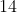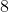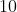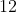Correct answer:Explanation: In setting up a word problem like this, be sure to use meaningful variables. Here your variables are lattes (which you can call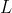) and coffees (which you can call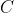). You can set up an equation to account for the$60 total: $6 times the number of lattes plus$3 times the number of coffees will account for the $60, so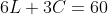. You can then simplify by dividing everything by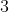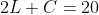. You can also set up an equation based on the fact that there were 2 more coffees than lattes. The number of coffees,, is equal to 2 plus the number of lattes,, so: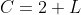With two equations and two variables, you can now solve. A quick way to do that is to subtractfrom both sides of the second equation: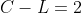If you then multiply both sides by 2, you have: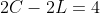Which sets up for the elimination method when you stack and add the two equations: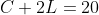This yields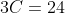, so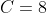. When you plug that back in to, you see that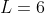, meaning that the total number of drinks is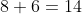. ### Example Question #23 : Solving Word Problems A certain bakery sells only cupcakes and brownies, and charges$5 for each cupcake and $4 for each brownie. If Jeremy spent$34 purchasing 8 items at the bakery, how many brownies did he purchase?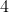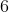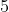Explanation:

Your job on this word translations problem is to assign variables (for cupcakes and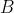for brownies) and then set up equations. You know that one equation is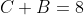, since the number of cupcakes plus the number of brownies must equal 8. The other equation deals with the price; Jeremy spends a total of $34, and that cost is made up of the number of cupcakes he buys times$5 for each, and the number of brownies he buys times $4 each brownie. So that equation is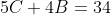. With those two equations, you should see that they stack up nicely so that you can use the Elimination Method to solve. You have:And then if you multiply the entire bottom equation by -4, you'll have: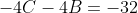If you then sum the equations, you're left with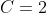. But remember: the question asked you about brownies, not cupcakes, so be careful with the temptation to select 2 as your answer! Ifand, that means that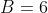. ### Example Question #1 : Solving Systems Of Equations In Word Problems A movie theater sold 120 tickets to the matinee showing of a popular children's movie, and 150 tickets to the evening showing. The theater sold the same number of adult tickets for each show, but for the evening show was just 20 children's tickets short of selling twice as many children's tickets as it did for the matinee. How many children's tickets were sold to the evening show? Possible Answers: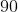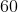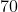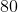Correct answer:Explanation: In this word problem, it's first helpful to assign meaningful variables. Since the matinee equation is the "cleaner" of the two, you might start with: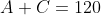Meaning that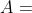the number of adult tickets to the matinee and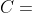the number of children's tickets to the matinee. Then look at the second equation. Since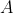is the same for each equation you're done there, but then unpack the relationship between matinee children's tickets and evening children's tickets. If, for the evening show, the number was "20 less than double," that means that you'd double the earlier number of children's tickets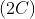and subtract 20 to get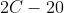. That means that your second equation is: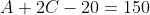So you have two equations now:So use the Elimination Method and subtract the simpler, matinee equation from the more-complex, evening equation and you'll be left with: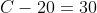So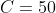But remember: C is the number of children's matinee tickets, so you'll need to convert to the number of children's evening tickets. That number is, which equals 80. ### Example Question #10 : Solving Systems Of Equations In Word Problems A sporting good store sells one type of baseball bat and one type of baseball. The cost for 2 bats and 4 balls is$160. The cost for 1 bat and 6 balls is $160, as well. If someone were to buy an equal number of bats and balls, at most how many bats can he purchase if he has a budget of$240 for the purchase?Explanation:

This word problem starts you off with two equations. If you assign variables as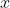= bats and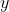= balls, you have: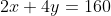, which reduces to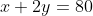and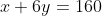You can then use the Elimination Method to eliminate theterms and solve for: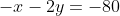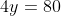, so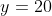. Plug that back in, and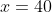. So now you have the prices.

At this point, you know that you need the same number ofand, and that the total can only be as high as \$240. If you call that same number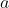, you have: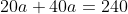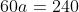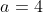. So you'll be able to buy 4 bats and 4 balls.

### All SAT Mathematics Resources